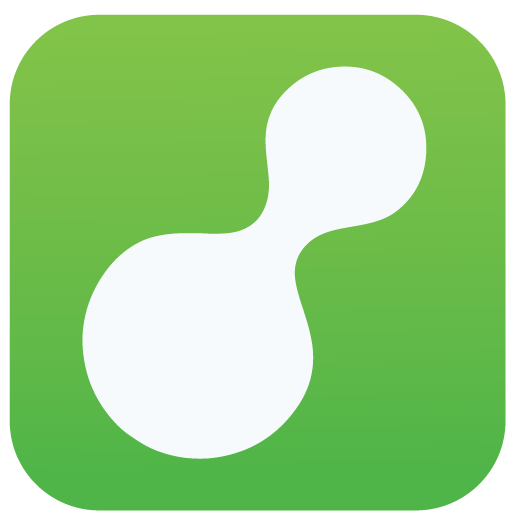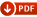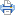# Volume conversion table and Calculator Chart#Volume Conversion Calculator

An online volume conversion instantly.

 Milliliters Liters Fluid ounces Pints Cups Quarts Gallons#Volume Conversion TableMeasurements EQ Equivalents
1 Tablespoon = 3 Teaspoons
1 Fluid Ounce = 2 Tablespoons
1 Cup = 8 Ounces
1 Pint = 2 Cups
1 Quart = 2 Pints
1 Gallon = 4 Quarts
1/4 Teaspoon = 1.25 Milliliters (mls.)
1/2 Teaspoon = 2.5 Milliliters (mls.)
1 Teaspoon = 5 Milliliters (mls.)
1 Tablespoon = 15 Milliliters (mls.)
1 Fluid Ounce = 30 Milliliters (mls.)
1/4 Cup = 60 Milliliters (mls.)
1/3 Cup = 78 Milliliters (mls.)
1/2 Cup = 118.2 Milliliters (mls.)
1 Cup = 236.5 Milliliters (mls.)
1 Pint (U.S.) = 473 Milliliters (mls.)
1 Pint (U.S.) = 28.875 Cubic Inches
1 Pint (U.S.) = 28.875 Cubic Inches
1 Pint (U.S.) = 16 Ounces
1 Pint (U.S.) = 0.4732 Liters
1 Quart (U.S.) = 946 Milliliters (mls.)
1 Quart (U.S.) = 4 Cups
1 Quart (U.S.) = 32 Ounces
1 Gallon = 128 Ounces
1 Gallon (U.S.) = 3.79 Liters
1 Gallon (U.S.) = 3785 Cubic Centimeters
1 Gallon = 4 Quarts
1 Gallon = 8 Pints
1 Liter = 1000 Cubic Centimeters
1 Liter = 1000 Milliliters (mls.)
1 Liter = 1.057 Quarts
1 Liter = 0.264 Gallons (U.S.)
1 Liter = 1.76 Pints
1 Liter = 35.2 Fluid Ounces

###More Calculators ►

Online math number calculation, formulas##Top Calculators ►

Online Algebra calculation, formulas , Digital calculation, Statistical calculation, Math Converters Pet Age Calculator,